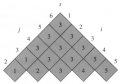# Matrix Chain Multiplication: How to determine optimal way of multiplying matrices?

#### zulfi100

Joined Jun 7, 2012
656
Hi,

I am studying Matrix chain Multiplication to find out the optimal way of multiplying a series of matrices so that we can reduce the number of multiplications. I have got this example from the book which multiplies the matrices having dimensions given below:

A1 30 * 35

A2 32 * 15

A3 15 * 5

A4 5 * 10

A5 10 * 20

A6 20 * 25

From these matrices, it creates the 2 tables m table & s table which are uploaded:MTM(A, s, I, j)

If j> i

Then X<-- MCM(A, s, I, s[I,j])

Y<-- MCM(A, s, s[I,j] + 1, j)

return MCM (X, Y)

else return Ai

They are invoking the algorithm by:

MCM(A, s, 1, 6)

Can some body guide me what is the value of A & s?
Later on i want to understand the ordering of matrix for chain multiplication.

Zulfi.